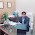1.       In a triangle    ABC , if A=120° , b=2 and C=30° then a=? a)3 b)2 √3 c)√3 d)2 2.       In    ABC , if a=5,b=12 and c=13, then the value of sin2B= ? a)      125/144 b)      120/169 c)       144/169 d)      None 3.       The roots of 2x^2-6x+7=0 are…. a)      Real , unequal and rational b)      Real , unequal and irrational c)       Real and equal d)      Imaginary 4.       The roots of 2x^2 -6x+3=0 are…. a)      Real , unequal and rational b)      Real , unequal and irrational c)       Real and equal d)      Imaginary 5.       The polynomial x^2-x+1 has – a)      One proper linear factor b)      Two proper linear factor c)       No proper linear factor d)      None of these 6.       The roots of the eqation 15x^2-28=x are a)      Real and equal b)      Real and unequal c)       Complex numbers d)      None of these 7.       The roots of ax^2+bx+c=0 will be reciprocal to each other, if a)      a=1/c b)      a=c c)       b=ac d)      a=b 8.       A die is thrown. What is the probability of getting a prime number ? a)      1/6 b)      1/3 c)       1/2 d)      1 9.       In simultaneous throw of two dice the probability of getting a total of 7 is : a)      7/36 b)      1/7 c)       1/4 d)      1/6 10.    A coin is tossed successively three times. The probability of getting one head or two heads is : a)      2/3 b)      3/4 c)       4/9 d)      None

1.2.1.